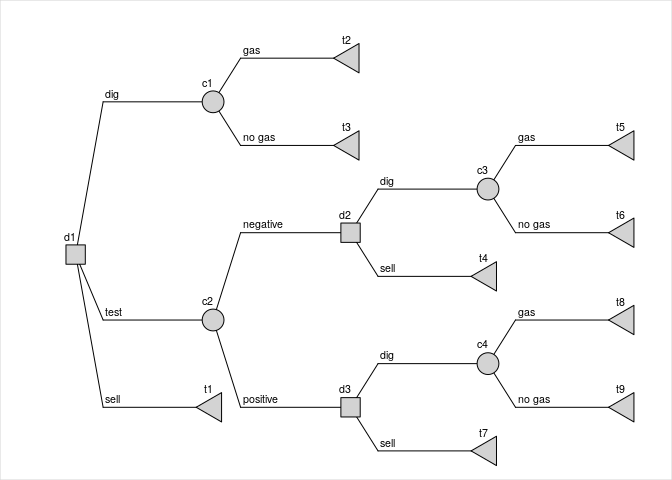# Introduction

Kaminski et al1 (Fig 7) provide an example of a decision tree with multiple decision nodes, including some that are descendants of another decision node. This vignette illustrates how rdecision can be used to model a complex decision tree, using the example from Figure 7 of Kamiński et al.1

# The problem

Kaminski et al1 state the problem as follows:

Consider an investor owning a plot of land, possibly (a priori probability amounting to 70%) hiding shale gas layers. The plot can be sold immediately (800, all prices in $’000). The investor can build a gas extraction unit for a cost of 300. If gas is found, the profit will amount to 2,500 (if not there will be no profit, and no possibility of selling the land). Geological tests can be performed for a cost of 50, and will produce either a positive or negative signal. The sensitivity amounts to 90% and the specificity amounts to 70%. The installation can be built after the test or the land may be sold for 1000 (600) after a positive (negative) test result. # Creating the model ## Constructing the tree The model, comprising three decision nodes, four chance nodes, nine leaf nodes and 15 edges, is constructed as follows. Costs, benefits and probabilities are associated with each edge, which must be an Action or a Reaction object, see figure.  # nodes d1 <- DecisionNode$new("d1")
d2 <- DecisionNode$new("d2") d3 <- DecisionNode$new("d3")
c1 <- ChanceNode$new("c1") c2 <- ChanceNode$new("c2")
c3 <- ChanceNode$new("c3") c4 <- ChanceNode$new("c4")
t1 <- LeafNode$new("t1") t2 <- LeafNode$new("t2")
t3 <- LeafNode$new("t3") t4 <- LeafNode$new("t4")
t5 <- LeafNode$new("t5") t6 <- LeafNode$new("t6")
t7 <- LeafNode$new("t7") t8 <- LeafNode$new("t8")
t9 <- LeafNode$new("t9") # probabilities p.sens <- 0.9 p.spec <- 0.7 p.gas <- 0.7 p.nogas <- 1-p.gas p.ptest <- p.sens*p.gas + (1-p.spec)*p.nogas p.ntest <- (1-p.sens)*p.gas + p.spec*p.nogas p.gas.ptest <- p.sens*p.gas / p.ptest p.gas.ntest <- (1-p.sens)*p.gas / p.ntest # edges E <- list( Action$new(d1,t1,"sell",benefit=800),
Action$new(d1,c1,"dig",cost=300), Reaction$new(c1,t2,p=p.gas,benefit=2500,label="gas"),
Reaction$new(c1,t3,p=p.nogas,label="no gas"), Action$new(d1,c2,"test",cost=50),
Reaction$new(c2,d2,p=p.ntest,label="negative"), Action$new(d2,t4,"sell",benefit=600),
Action$new(d2,c3,"dig",cost=300), Reaction$new(c3,t5,p=p.gas.ntest,benefit=2500,label="gas"),
Reaction$new(c3,t6,p=(1-p.gas.ntest),label="no gas"), Reaction$new(c2,d3,p=p.ptest,label="positive"),
Action$new(d3,t7,"sell",benefit=1000), Action$new(d3,c4,"dig",cost=300),
Reaction$new(c4,t8,p=p.gas.ptest,benefit=2500,label="gas"), Reaction$new(c4,t9,p=(1-p.gas.ptest),label="no gas")
)
# tree
V <- list(d1,d2,d3, c1,c2,c3,c4, t1,t2,t3,t4,t5,t6,t7,t8,t9)
DT<-DecisionTree$new(V,E) ## Tree diagramDecision tree used in the shale gas problem # Evaluating the strategies There are a total of 12 possible strategies (3 choices from node d1 $$\times$$ 2 choices at node d2 $$\times$$ 2 choices at node d3). But some of these are not unique. For example if the choice at node d1 is “sell”, the choices at nodes d2 and d3 (4 possible combinations) are unimportant; all four such strategies are identical. Method evaluate calculates the expected cost, benefit and utility of each traversable path for each strategy, and aggregates by strategy. The results for the gas problem are computed as follows. Pay-off is defined as benefit minus cost. # find optimal strategies RES <- DT$evaluate()
RES$Payoff <- RES$Benefit-RES\$Cost

This gives the following pay-off for each strategy:

d1 d2 d3 Cost Benefit Payoff
dig dig dig 300 1750 1450
sell dig dig 0 800 800
test dig dig 350 1750 1400
dig sell dig 300 1750 1450
sell sell dig 0 800 800
test sell dig 266 1743 1477
dig dig sell 300 1750 1450
sell dig sell 0 800 800
test dig sell 134 895 761
dig sell sell 300 1750 1450
sell sell sell 0 800 800
test sell sell 50 888 838

The optimal strategy is test/sell/dig, i.e. test, sell if negative and dig otherwise. The expected pay-off from this strategy is 1477.